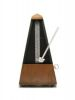# Music for Kids

## What is a musical Note?The term "note" in music describes the pitch and the duration of a musical sound.

What is the Pitch of a Musical Note?

The pitch describes how low or high a note sounds. Sound is made up of vibrations or waves. These waves have a speed or frequency that they vibrate at. The pitch of the note changes depending on the frequency of these vibrations. The higher the frequency of the wave, the higher the pitch of the note will sound.

What is the Musical Scale and the Note Letters?

In music there are specific pitches that make up standard notes. Most musicians use a standard called the chromatic scale. In the chromatic scale there are 7 main musical notes called A, B, C, D, E, F, and G. They each represent a different frequency or pitch. For example, the "middle" A note has a frequency of 440 Hz and the "middle" B note has a frequency of 494 Hz.

There are variations of each of these notes called the sharp and the flat. A sharp is one half step up and a flat is one half step down. For example, a half step up from C would be the C-sharp.

What is an Octave?

After the note G, there is another set of the same 7 notes just higher. Each set of these 7 notes and their half step notes is called an octave. The "middle" octave is often called the 4th octave. So the octave below in frequency would be the 3rd and the octave above in frequency would be the 5th.

Each note in an octave is twice the pitch or frequency of the same note in the octave below. For example, an A in the 4th octave, called A4, is 440Hz and an A in the 5th octave, called A5 is 880Hz.Duration of a Musical Note

The other important part of a musical note (besides pitch) is the duration. This is the time that the note is held or played. It is important in music that notes are played in time and rhythm. Timing and meter in music is very mathematical. Each note gets a certain amount of time in a measure.

For example, a quarter note would be played for 1/4 of the time (or one count) in a 4 beat measure while a half note would be played for 1/2 the time (or two counts). A half note is played twice as long as a quarter note.Activities